Normal view

# Applied logistic regression / David W. Hosmer.

Material type:TextLanguage: English Publisher: New Jersy : Wiley, 2013Edition: 3rd edDescription: xvi, 500 p. : ill. ; 25 cmISBN: 9780470582473 (hardback); 0470582472DDC classification: 519.536 LOC classification: QA278.2 | .H67 2013Other classification: MAT029030 Online resources: WorldCat details | Ebook Fulltext
Contents:
Table of contents Applied Logistic Regression; Contents; Preface to the Third Edition; 1 Introduction to the Logistic Regression Model; 1.1 Introduction; 1.2 Fitting the Logistic Regression Model; 1.3 Testing for the Significance of the Coefficients; 1.4 Confidence Interval Estimation; 1.5 Other Estimation Methods; 1.6 Data Sets Used in Examples and Exercises; 1.6.1 The ICU Study; 1.6.2 The Low Birth Weight Study; 1.6.3 The Global Longitudinal Study of Osteoporosis in Women; 1.6.4 The Adolescent Placement Study; 1.6.5 The Burn Injury Study; 1.6.6 The Myopia Study; 1.6.7 The NHANES Study. 1.6.8 The Polypharmacy StudyExercises; 2 The Multiple Logistic Regression Model; 2.1 Introduction; 2.2 The Multiple Logistic Regression Model; 2.3 Fitting the Multiple Logistic Regression Model; 2.4 Testing for the Significance of the Model; 2.5 Confidence Interval Estimation; 2.6 Other Estimation Methods; Exercises; 3 Interpretation of the Fitted Logistic Regression Model; 3.1 Introduction; 3.2 Dichotomous Independent Variable; 3.3 Polychotomous Independent Variable; 3.4 Continuous Independent Variable; 3.5 Multivariable Models; 3.6 Presentation and Interpretation of the Fitted Values. 3.7 A Comparison of Logistic Regression and Stratified Analysis for 2 x 2 TablesExercises; 4 Model-Building Strategies and Methods for Logistic Regression; 4.1 Introduction; 4.2 Purposeful Selection of Covariates; 4.2.1 Methods to Examine the Scale of a Continuous Covariate in the Logit; 4.2.2 Examples of Purposeful Selection; 4.3 Other Methods for Selecting Covariates; 4.3.1 Stepwise Selection of Covariates; 4.3.2 Best Subsets Logistic Regression; 4.3.3 Selecting Covariates and Checking their Scale Using Multivariable Fractional Polynomials; 4.4 Numerical Problems; Exercises. 5 Assessing the Fit of the Model5.1 Introduction; 5.2 Summary Measures of Goodness of Fit; 5.2.1 Pearson Chi-Square Statistic, Deviance, and Sum-of-Squares; 5.2.2 The Hosmer-Lemeshow Tests; 5.2.3 Classification Tables; 5.2.4 Area Under the Receiver Operating Characteristic Curve; 5.2.5 Other Summary Measures; 5.3 Logistic Regression Diagnostics; 5.4 Assessment of Fit via External Validation; 5.5 Interpretation and Presentation of the Results from a Fitted Logistic Regression Model; Exercises; 6 Application of Logistic Regression with Different Sampling Models; 6.1 Introduction. 6.2 Cohort Studies6.3 Case-Control Studies; 6.4 Fitting Logistic Regression Models to Data from Complex Sample Surveys; Exercises; 7 Logistic Regression for Matched Case-Control Studies; 7.1 Introduction; 7.2 Methods For Assessment of Fit in a 1-M Matched Study; 7.3 An Example Using the Logistic Regression Model in a 1-1 Matched Study; 7.4 An Example Using the Logistic Regression Model in a 1-M Matched Study; Exercises; 8 Logistic Regression Models for Multinomial and Ordinal Outcomes; 8.1 The Multinomial Logistic Regression Model. 8.1.1 Introduction to the Model and Estimation of Model Parameters.
Summary: Summary: "A new edition of the definitive guide to logistic regression modeling for health science and other applicationsThis thoroughly expanded Third Edition provides an easily accessible introduction to the logistic regression (LR) model and highlights the power of this model by examining the relationship between a dichotomous outcome and a set of covariables. Applied Logistic Regression, Third Edition emphasizes
Tags from this library: No tags from this library for this title.Average rating: 0.0 (0 votes)
Item type Current location Collection Call number Copy number Status Date due Barcode Item holdsE-Book
E-book
Non-fiction 519.536 HOA 2013 (Browse shelf) Not for loanText
Reserve Section
Non-fiction 519.536 HOA 2013 (Browse shelf) C-1 Available 26911
Total holds: 0
##### Browsing EWU Library shelves, Shelving location: Reserve Section Close shelf browser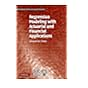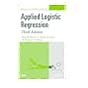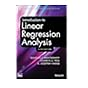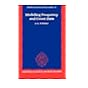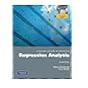519.536 FRG 2010 Regression modeling with actuarial and financial applications / 519.536 HOA 2013 Applied logistic regression. 519.536 HOA 2013 Applied logistic regression. 519.536 HOA 2013 Applied logistic regression / 519.536 INT 2012 Introduction to linear regression analysis / 519.536 LIM 1995 Modelling frequency and count data / 519.536 MES 2012 A second course in statistics :

Print version:
Hosmer, David W.
Applied logistic regression.
Hoboken, New Jersey : Wiley, 
(DLC) 2013000403

Includes bibliographical references and index.

Table of contents Applied Logistic Regression; Contents; Preface to the Third Edition; 1 Introduction to the Logistic Regression Model; 1.1 Introduction; 1.2 Fitting the Logistic Regression Model; 1.3 Testing for the Significance of the Coefficients; 1.4 Confidence Interval Estimation; 1.5 Other Estimation Methods; 1.6 Data Sets Used in Examples and Exercises; 1.6.1 The ICU Study; 1.6.2 The Low Birth Weight Study; 1.6.3 The Global Longitudinal Study of Osteoporosis in Women; 1.6.4 The Adolescent Placement Study; 1.6.5 The Burn Injury Study; 1.6.6 The Myopia Study; 1.6.7 The NHANES Study. 1.6.8 The Polypharmacy StudyExercises; 2 The Multiple Logistic Regression Model; 2.1 Introduction; 2.2 The Multiple Logistic Regression Model; 2.3 Fitting the Multiple Logistic Regression Model; 2.4 Testing for the Significance of the Model; 2.5 Confidence Interval Estimation; 2.6 Other Estimation Methods; Exercises; 3 Interpretation of the Fitted Logistic Regression Model; 3.1 Introduction; 3.2 Dichotomous Independent Variable; 3.3 Polychotomous Independent Variable; 3.4 Continuous Independent Variable; 3.5 Multivariable Models; 3.6 Presentation and Interpretation of the Fitted Values. 3.7 A Comparison of Logistic Regression and Stratified Analysis for 2 x 2 TablesExercises; 4 Model-Building Strategies and Methods for Logistic Regression; 4.1 Introduction; 4.2 Purposeful Selection of Covariates; 4.2.1 Methods to Examine the Scale of a Continuous Covariate in the Logit; 4.2.2 Examples of Purposeful Selection; 4.3 Other Methods for Selecting Covariates; 4.3.1 Stepwise Selection of Covariates; 4.3.2 Best Subsets Logistic Regression; 4.3.3 Selecting Covariates and Checking their Scale Using Multivariable Fractional Polynomials; 4.4 Numerical Problems; Exercises. 5 Assessing the Fit of the Model5.1 Introduction; 5.2 Summary Measures of Goodness of Fit; 5.2.1 Pearson Chi-Square Statistic, Deviance, and Sum-of-Squares; 5.2.2 The Hosmer-Lemeshow Tests; 5.2.3 Classification Tables; 5.2.4 Area Under the Receiver Operating Characteristic Curve; 5.2.5 Other Summary Measures; 5.3 Logistic Regression Diagnostics; 5.4 Assessment of Fit via External Validation; 5.5 Interpretation and Presentation of the Results from a Fitted Logistic Regression Model; Exercises; 6 Application of Logistic Regression with Different Sampling Models; 6.1 Introduction. 6.2 Cohort Studies6.3 Case-Control Studies; 6.4 Fitting Logistic Regression Models to Data from Complex Sample Surveys; Exercises; 7 Logistic Regression for Matched Case-Control Studies; 7.1 Introduction; 7.2 Methods For Assessment of Fit in a 1-M Matched Study; 7.3 An Example Using the Logistic Regression Model in a 1-1 Matched Study; 7.4 An Example Using the Logistic Regression Model in a 1-M Matched Study; Exercises; 8 Logistic Regression Models for Multinomial and Ordinal Outcomes; 8.1 The Multinomial Logistic Regression Model. 8.1.1 Introduction to the Model and Estimation of Model Parameters.

Summary:
"A new edition of the definitive guide to logistic regression modeling for health science and other applicationsThis thoroughly expanded Third Edition provides an easily accessible introduction to the logistic regression (LR) model and highlights the power of this model by examining the relationship between a dichotomous outcome and a set of covariables. Applied Logistic Regression, Third Edition emphasizes

Applied Statistics

There are no comments for this item.

to post a comment.
Share

Library Home | Contacts | E-journals
Copyright @ 2011-2019 EWU Library
East West University